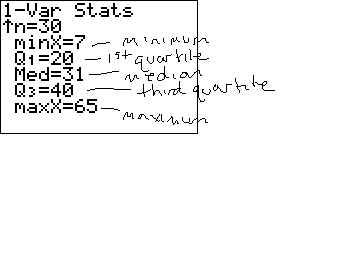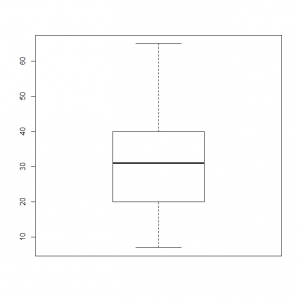# How to Make a Box plot (Box and Whiskers Plot) By Hand

Typically, statisticians are going to use software to help them look at data using a box plot. However, when you are first learning about box plots, it can be helpful to learn how to sketch them by hand. This way, you will be very comfortable with understanding the output from a computer or your calculator. In the following lesson, we will look at the steps needed to sketch boxplots from a given data set.

## Example data

Remember, the goal of any graph is to summarize a data set. There are many possible graphs that one can use to do this. One of the more common options is the histogram, but there are also dotplots, stem and leaf plots, and as we are reviewing here – boxplots (which are sometimes called box and whisker plots). Like a histogram, box plots ignore information about each individual data value and instead show the overall pattern.

To review the steps, we will use the data set below. Let’s suppose this data set represents the salaries (in thousands) of a random sample of employees at a small company.

 714141416 1820202123 2727272931 3132323436 4040404040 4251566065

## Steps to Making Your Box plot

### Step 1: Calculate the five number summary for your data set

The five number summary consists of the minimum value, the first quartile, the median, the third quartile, and the maximum value. While these numbers can also be calculated by hand (here is how to calculate the median by hand for instance), they can quickly be found on a TI83 or 84 calculator under 1-varstats. The video below shows you how to get to that menu on the TI84:

For this data set, you will get the following output:### Step 2: Identify outliers

Other than “a unique value”, there is not ONE definition across statistics that is used to find an outlier. As you study statistics, you will see that different settings will use different techniques to flag or mark a potential outlier. With boxplots, this is done using something called “fences”. The idea is that anything outside the fences is a potential outlier and shouldn’t be included in the main group that we graph. Instead it will be marked with a asterisk or other symbol.

#### The lower fence

Any data value smaller than the lwoer fence will be considered an outlier. The lower fence is defined by the following formula:

$$\text{lower fence} = Q_{1} – 1.5(IQR)$$

This formula makes use of the IQR, or interquartile range. This is defined as:

$$\text{IQR} = Q_3 – Q_1$$

Using the calculator output, we have for this data set $$Q_1 = 20$$ and $$Q_3 = 40$$. This gives us:

\begin{align} \text{IQR} &= Q_{3}-Q_{1}\\ &= 40 – 20\\ &= 20\end{align}

and using this value:

\begin{align} \text{lower fence} &= Q_{1} – 1.5(IQR) \\ &= 20 -1.5(20)\\ &= 20 – 30\\ &= -10\end{align}

Since there are no values in the data set that are less than -10, there are no lower (small) outliers.

#### The upper fence

Similar to the lower fence, anything data value larger than the upper fence will be considered an outlier. This is defined by the following formula.

$$\text{upper fence} = Q_{3} + 1.5(IQR)$$

Using the calculation above, we know that $$\text{IQR} = 20$$. We also had $$Q_3 = 40$$. Therefore:

\begin{align}\text{upper fence} &= Q_{3} + 1.5(IQR)\\ &= 40 + 1.5(20) \\ &=40 + 30\\ &= 70\end{align}

The largest value in the data set is 65, so this means there is no upper (large) outlier.

Since there were no small or large outliers in the set, we can conclude there are no outliers overall.

### Step 3: Sketch the box plot using the model below

The main part of the box plot will be a line from the smallest number that is not an outlier to the largest number in our data set that is not an outlier. If a data set doesn’t have any outliers (like this one), then this will just be a line from the smallest value to the largest value. The rest of the plot is made by drawing a box from $$Q_{1}$$ to $$Q_{3}$$ with a line in the middle for the median. As a general example:

Additionally, if you are drawing your box plot by hand you must think of scale. In this data set, the smallest is 7 and the largest is 65. So starting the scale at 5 and counting by 5 up to 65 or 70 would probably give a nice picture. Then, since none of these are outliers, we will draw a line from 7, which is the smallest data value to 65, which is the largest data value. Finally, we will add a box from our quartiles ($$Q_1 = 20$$ and $$Q_3 = 40$$) and a line at the median of 31. All together we have:Of course, a software version will look quite a bit better. Also note that boxplots can be drawn horizontally or vertically and you may run across either as you continue your studies. As an example, here is the same boxplot done with R (a statistical software program) instead:## Summary

Remember – pay attention to how these box plots are put together in order to do a better job at reading the information they provide. Since you now know that middle line is the median, you can just look at the box plot and know that 50% of the salaries were less than \$31,000 or so. As you can see, a box plot can not only show you the overall pattern but also contains a lot of information about the data set. To see more about the information you can gather from a boxplot, see: How to read a boxplot